# Lesson 4: An Engineering Introduction to Machines–Pulleys (December 13 & 14)

Objectives: (1) To introduce students to the basic concepts of machines, as used by engineers; and (2) to specifically learn the most basic principles of the PULLEY.

INSTRUCTIONS:

• Read the article below.
• Answer the questions in the blue boxes. PLEASE NOTE: There are 20 questions in blue boxes inserted throughout the article.

Based on Mr. Tunon’s notes and powerpoint1

REVIEW: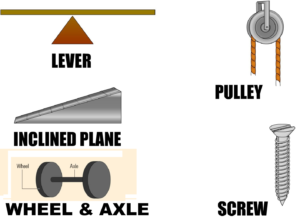Types of machines

In Engineering, there are five main types of simple machines:

• levers,
• wheels and axles (which count as one),
• pulleys,
• Incline planes, and
• screws.

A machine is an object or mechanical device that receives an input amount of work and transfers the energy to an output amount of work. For an ideal machine, the input work and output work are always the same. Remember that work is force times distance; even though the work input and output are equal, the input force does not necessarily equal the output force, nor does the input distance necessarily equal the output distance.2

QUESTION 1 out of 20 (Write the question and answer):  For an ideal machine, the input work and output work are always the same.  (TRUE or FALSE?)

QUESTION 2 out of 20 (Write the question and answer):  Increasing the number of wheels decreases the amount of friction.  (TRUE or FALSE?)

## PULLEY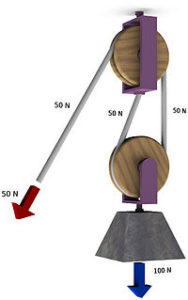Pulley – FIGURE No. 1

At first glance the pulley, and its variations, seems more like magic, than a simple machine.  But let’s take a closer look…

• Put two or more wheels together and loop a rope around them several times and you create a powerful lifting machine called a pulley. Each time the rope wraps around the wheels, you create more lifting power or mechanical advantage. If there are four wheels and the rope wraps around them, the pulley works as though four ropes are supporting the load. So you can lift four times as much!

QUESTION 3 out of 20 (Incorporate the question in your answer):  Write a definition for the simple machine called PULLEY.

QUESTION 4 out of 20 (Incorporate the question in your answer):  In a pulley, what is the relationship/ratio between the  number of wheels and the lifting power?

### How pulleys work

If you have a single wheel and a single rope, a pulley helps you reverse the direction of your lifting force. So, as in the picture below, you pull the rope down to lift the weight up. If you want to lift something that weighs 100kg, you have to pull down with a force equivalent to 100kg.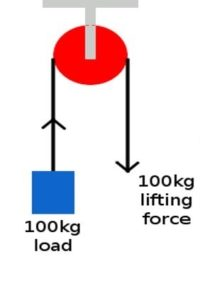QUESTION 5 out of 20 (Incorporate the question in your answer):   If there is no mechanical advantage to a one wheel pulley (that is, input force equals output force), then why would you use one?  Give an example of an application in which a one wheel pulley would make sense?

• Now if you add more ropes and wheels, you can reduce the effort you need to lift the weight. Suppose you have two wheels and two ropes, arranged as in the figure below. The 100kg weight is now supported by two ropes instead of one, so you can lift it by pulling with a force of just 50kg—half as much! That’s why we say a pulley with two wheels and ropes gives a mechanical advantage (ME) of two.

QUESTION 6 out of 20 (Incorporate the question in your answer): Look carefully at the graphic at the top of the PULLEY section.  The caption is, “Pulley – FIGURE No. 1.”  When the various descriptions of pulleys say something like,   “The 100kg weight is now supported by two ropes instead of one…” (see bullet point above this box), does this mean you have to physically cut the longer rope into two separate ropes to make the pulley work?  Answer yes or  no, and explain why.

• Mechanical advantage is a measurement of how much a simple machine multiples a force. The bigger the mechanical advantage, the less force you need.
• With two wheels and two ropes, a pulley halves the lifting force you need. But you have to pull the end of the rope twice as far.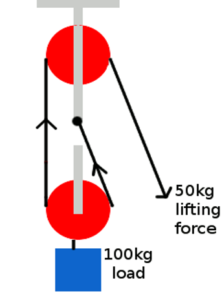QUESTION 7 out of 20 (Incorporate the question in your answer):   Is it possible to have an odd number of wheels in a pulley system?

• Various multiple wheel combinations.  See the graphic below, showing one through four wheels.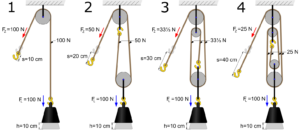pulley systems with one to four wheels
• What if you use four wheels held together by a long rope that loops over them, as in the picture below? You can see that the 100kg weight is now supported by four ropes on the left (ignoring the end of the rope you’re pulling with). That means each rope is supporting a quarter of the total 100kg weight, or 25kg, and to raise the weight into the air, you have to pull with only a quarter of the force—also 25kg.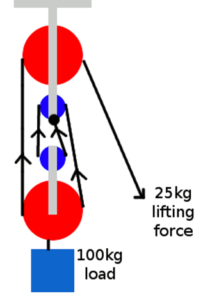QUESTION 8 out of 20 (Incorporate the question in your answer):  In the pulley pictured just above (four wheels) what force would be necessary to lift a 500 pound load.  EXPLAIN how you arrived at your answer.  Assume an ideal (no friction) machine.

• We say a pulley with four wheels and ropes gives a mechanical advantage of four. That’s twice as good as a pulley with two ropes and wheels.

QUESTION 9 out of 20 (Incorporate the question in your answer):  EXPLAIN what the term “mechanical advantage” means.

• With four wheels and four ropes, a pulley cuts the lifting force you need to one quarter. But you have to pull the end of the rope four times as far.

Block & Tackle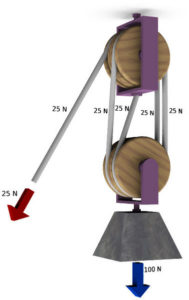• block and tackle
• A block and tackle is a series of pulleys that are connected by a rope or cord and used to lift heavy objects easier and more efficiently.
• The pulleys are put together in a way so it looks like a block.  “Tackle” is a nautical term, referring to the rigging and apparatus of a ship
• In each block and tackle there is at least one fixed pulleys, meaning they stay at that point and doesn’t move up or down when used, and at least one moveable pulley that when used moves up or down.
• It is used so you can trade force for distance. Pulling a 100 pound block strait up with one pulley means that you will have to apply 100 pounds of force to keep it suspended.
• If you add another pulley to the machine, the pulley is still lifting 100 pounds, but the weight is distributed between two pulleys (50 pounds) and you can use less force to pull on the load in the same amount of distance.

QUESTION 10 out of 20 (Incorporate the question in your answer):  DEFINE “block and tackle” pulley system.

QUESTION 11 out of 20 ( Copy the question and fill in the blank):  A “block and tackle” pulley system can trade __________ for distance.

QUESTION 12 out of 20 (Incorporate the question in the answer):  Why is it called a “block and tackle” pulley system?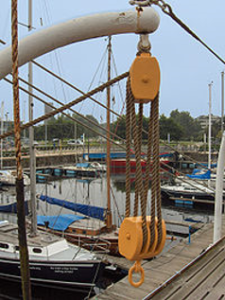block and tackle
• The more pulleys you use the less force is needed to lift the object.
• A block and tackle can have as many pulleys on it as you want, but the more pulleys there is the more friction there is going to be.

• Advantages to pulling the rope in the direction of the load being moved is that is pulling on the moveable pulleys not the fixed ones and has a better chance of staying together.

• QUESTION 13 out of 20 ( Copy the question and fill in the blank):   The more pulleys you use the less ________ is required.
• QUESTION 14 out of 20 (Write the question and answer):  Generally speaking, the more pulleys you use the less force is needed to lift the object.  (TRUE or FALSE?)

What’s the catch?

• If you raise a weight of 100kg a distance of 1 meter off the ground, you have to do the same amount of work whether you use a pulley or not: you have to move the same force over the same distance. If you use a pulley and reduce the force you’re using by a quarter, you still have to do the same amount of work. It’s just that you have to pull the end of the rope four times further to make each of the four supporting ropes rise by the same amount.
• That’s the catch with a pulley. You pull with less force, but you have to pull further (and, generally speaking, use the force for longer). Far from using less energy with a pulley, you actually have to use a little bit more because of the friction where the ropes rub against the pulley wheels.

The Belt and Pulley (not the same as the simple machine, but interesting nonetheless)

• Pulleys are used to change the speed, direction of rotation, or turning force or torque.3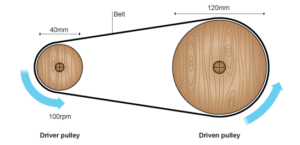• belt and pulley system
• A pulley system consists of two pulley wheels each on a shaft, connected by a belt. This transmits rotary motion and force from the input, or driver shaft, to the output, or driven shaft.
• Assuming no slippage, the length of the belt does not impact the speed or rotation of the of the belt and pulley system.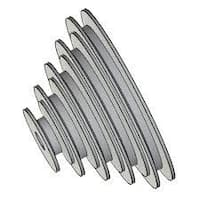• variable speed pulley (side view)
• If the pulley wheels are different sizes, the smaller one will spin faster than the larger one. The difference in speed is called the velocity ratio. This is calculated using the formula:
• Velocity ratio = diameter of the driven pulley ÷ diameter of the driver pulley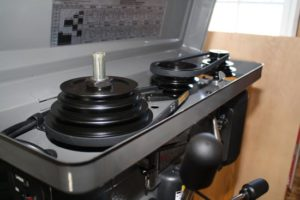variable speed pulleys installed to change the speed of the bit in a drill press
• If you know the velocity ratio and the input speed of a pulley system, you can calculate the output speed using the formula:
Output speed = input speed ÷ velocity ratio

QUESTION 15 out of 20 ( Copy the question and fill in the blank):   If the pulley wheels are different sizes, the smaller one will spin _______________ than the larger one.

• Another interesting variation of the belt and pulley system is the crossed belt, used to change the direction of rotation, as shown below.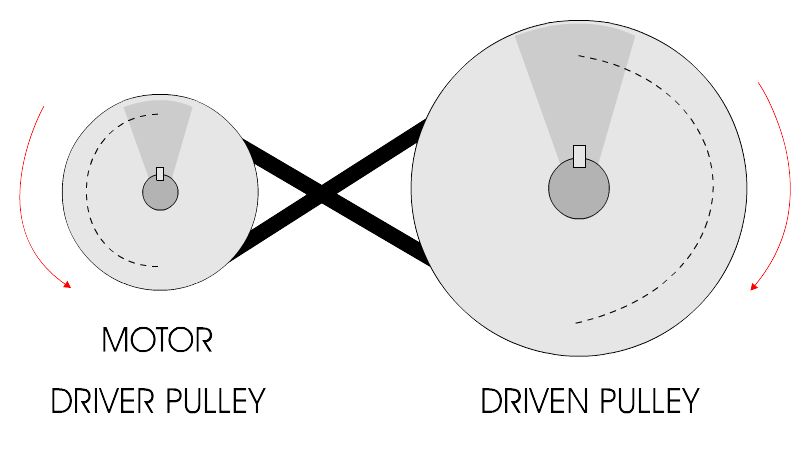Belt crossed to change the direction of rotation of the pulleys. Driver (left) is counter-clockwise. Driven pulley (right) is clockwise.

QUESTION 16 out of 20 (Write the question and answer):  How to you reverse the direction of the pulleys in a belt and pulley system?

QUESTION 17 out of 20 (Write the question and answer):  Does the length of the belt affect he speed or direction of the pulleys?  (Yes or No).

Draw a sketch of the following:

QUESTION  18 out of 20:  (sketch) A pulley system with two wheels. Label these parts: (a) pulleys, (b) rope, (c) weight being lifted.

QUESTION 19 out of 20:  (sketch)   A block and tackle system with two wheels. Label these parts: (a) pulleys, (b) rope, (c) weight being lifted.

QUESTION 20 out of 20:   (sketch) A belt and pulley system with two wheels. Label these parts: (a) pulleys, (b) belt, (c) direction of both pulleys.

1.   John Tunon J. P. Taravella High School
2. CK-12 website (https://www.ck12.org/physics/simple-machines-1501903848.65/lesson/Simple-Machines-PHYS/)
3.   BBC website (http://www.bbc.co.uk/schools/gcsebitesize/design/systemscontrol/mechanismsrev7.shtml)